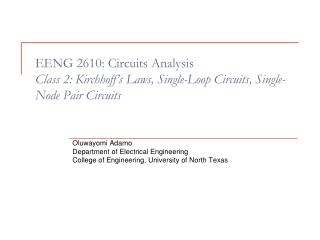DownloadDownload PresentationOluwayomi Adamo Department of Electrical Engineering

Oluwayomi Adamo Department of Electrical Engineering

Download PresentationOluwayomi Adamo Department of Electrical Engineering

- - - - - - - - - - - - - - - - - - - - - - - - - - - E N D - - - - - - - - - - - - - - - - - - - - - - - - - - -
Presentation Transcript

1. EENG 2610: Circuits AnalysisClass 2: Kirchhoff’s Laws, Single-Loop Circuits, Single-Node Pair Circuits Oluwayomi Adamo Department of Electrical Engineering College of Engineering, University of North Texas

2. Lumped-Parameter Circuit Wires in circuits are assumed perfect conductor Interconnections in circuits have zero resistance Wire doesn’t consume energy; energy in circuits is lumped in each circuit element. Node A point of connection of two or more circuit elements. A node is one end of a circuit element together with all the perfect conductor that are attached to it. Loop A loop is any closed path through the circuit in which no node is encountered more than once. Branch A branch is a portion of a circuit containing onlya single element and the nodes at each end of the element. Some Important Concepts

3. Kirchhoff’s Current Law (KCL) • KCL • The algebraic sum of the currents ENTERING any node is zero: Our sign convention for KCL:- The algebraic sign of the current is ‘plus’ if the current is entering the node- The algebraic sign of the current is ‘minus’ if the current is leaving the node Example 2.5: Write all KCL equations

4. Closed Surface as Super-Node • Super-Node • If some set of elements are completely contained within a surface that is interconnected, the surface is called super-node. • Generalized KCL for Super-Node • The algebraic sum of the currents entering any closed surface (or super-node) is zero. Write KCL equations for super-nodes

5. Kirchhoff’s Voltage Law (KVL) • KVL • The algebraic sum of the voltages around any loop is zero: • Voltage is defined as the difference in energy level of a unit positive charge located at each of the two points. KVL is based on the conservation of energy: the work required to move a unit charge around any loop is zero. • Our Sign Convention for KVL • As moving around a loop, the algebraic sign of voltage is positive in KVL equation if encounter the plus sign first, and the algebraic sign of voltage is negative in KVL equation if encounter the minus sign first.

6. = 18 V = 12 V Example 2.9: Using KVL equation to find

7. Double-subscript notation Vab = Va – Vb + and – notation Arrow notation Use an arrow between two points, pointing from negative node to positive node. KVL can be applied to a closed path even if part of the closed path is the arrow notation. a Vab V = Vab b Convention for Voltage Notation

8. Example 2.11: Use KVL to find Vae and Vec

9. Single-loop circuits Elements are connected in series. All elements carry same current. Voltage Divider Source v(t) is divided between two resistors in proportion to their resistance Single-Loop Circuits

10. + _ Multiple Voltage Sources in Single-Loop Circuit Equivalent Transform + _

11. Multiple Resistors in Single-Loop Circuit Equivalent Transform

12. Single-Node Pair Circuits • Single-Node Pair Circuits • Elements are connected in parallel • Elements have the same voltage across them • Current division

13. Multiple Current Sources in Single-Node Pair Circuits Equivalent Transform

14. + _ Multiple Resistors in Single-Node Pair Circuits Equivalent Transform General current divider:

15. Example 2.19: Find the current IL Equivalent circuit